# Do operator

The do operator is used in causal inference to denote an intervention. Given random variables$X,Y$, we write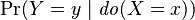$\Pr(Y=y \mid \mathit{do}(X=x))$ to mean the probability that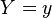$Y=y$ given we intervene and set$X$ to be$x$. In some texts, this is abbreviated to$\Pr(y\mid\hat x)$ (this notation assumes that the random variables corresponding to the individual values are clear from context). The notation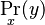$\Pr_x(y)$ is also used.
In general$\Pr(Y=y \mid \mathit{do}(X=x))$ is not the same as conditioning on$X=x$, i.e.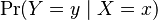$\Pr(Y=y \mid X=x)$. Note also that in the expression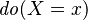$\mathit{do}(X=x)$, the subexpression$X=x$ does not mean the event where the random variable$X$ takes on the value$x$, i.e. the event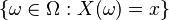$\{\omega\in\Omega : X(\omega) = x\}$. Thus, inside a do operator, the standard notational convention of probability theory does not hold. To stress the point, suppose the event$X=x$ can be specified in another way, such as by the event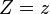$Z=z$. In this case, since$X=x$ and$Z=z$ are exactly the same set, the probabilities involving them, such as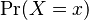$\Pr(X=x)$ vs$\Pr(Z=z)$ and$\Pr(Y=y \mid X=x)$ vs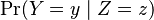$\Pr(Y=y \mid Z=z)$, should all be the same, but I don't think$\Pr(Y=y \mid \mathit{do}(X=x))$ and$\Pr(Y=y \mid \mathit{do}(Z=z))$ need be the same (check this).
Pearl: "An equivalent notation, using$\mathit{set}(x)$ instead of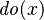$\mathit{do}(x)$, was used in Pearl (1995a). The$\mathit{do}(x)$ notation was first used in Goldszmidt and Pearl (1992) and is gaining in popular support. Lauritzen (2001) used$P ( y \mid X \leftarrow x)$. The expression$P(y \mid \mathit{do}(x))$ is equivalent in intent to$P(Y_x = y)$ in the potential-outcome model introduced by Neyman (1923) and Rubin (1974) and to the expression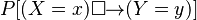$P[(X = x) \mathbin{\Box\!\!\rightarrow} (Y = y)]$ in the counter-factual theory of Lewis (1973b)."